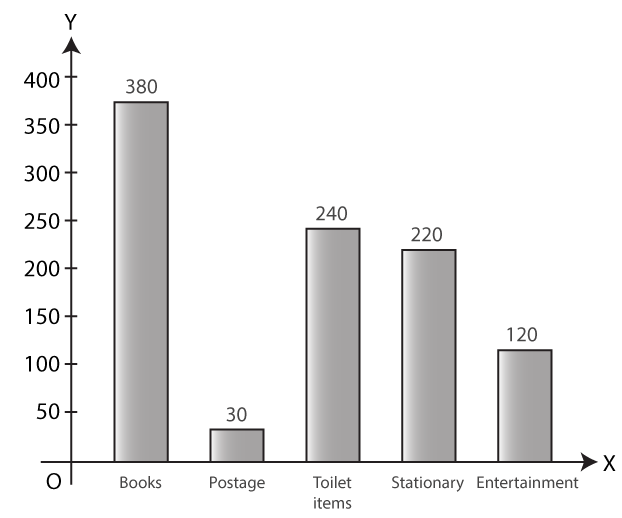# Selina Solutions Concise Mathematics Class 6 Chapter 33 Data Handling

Selina Solutions Concise Mathematics Class 6 Chapter 33 Data Handling provides students with the technique of handling the given set of data. The collection of information in numerical form is called data. The solutions are created by the subject matter experts, keeping in mind the understanding capacity of students. Referring to these solutions, students improve their time management skill, which is important from an exam point of view. In order to score more marks, students can make use of Selina Solutions Concise Mathematics Class 6 Chapter 33 Data Handling PDF, from the links given here.

Chapter 33 deals with study of data in the form of frequency distribution table, pictograph and bar graph to represent the given set of data. The solutions are available in both chapter wise and exercise wise PDF format, as per the student’s requirements.

## Selina Solutions Concise Mathematics Class 6 Chapter 33 Data Handling Download PDF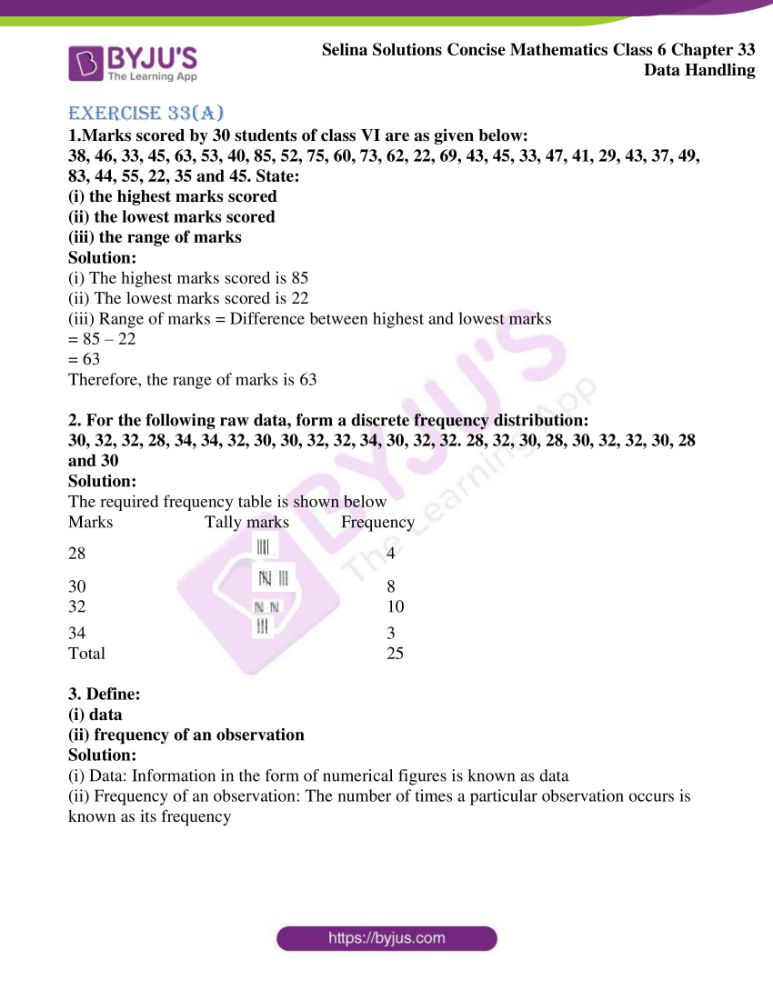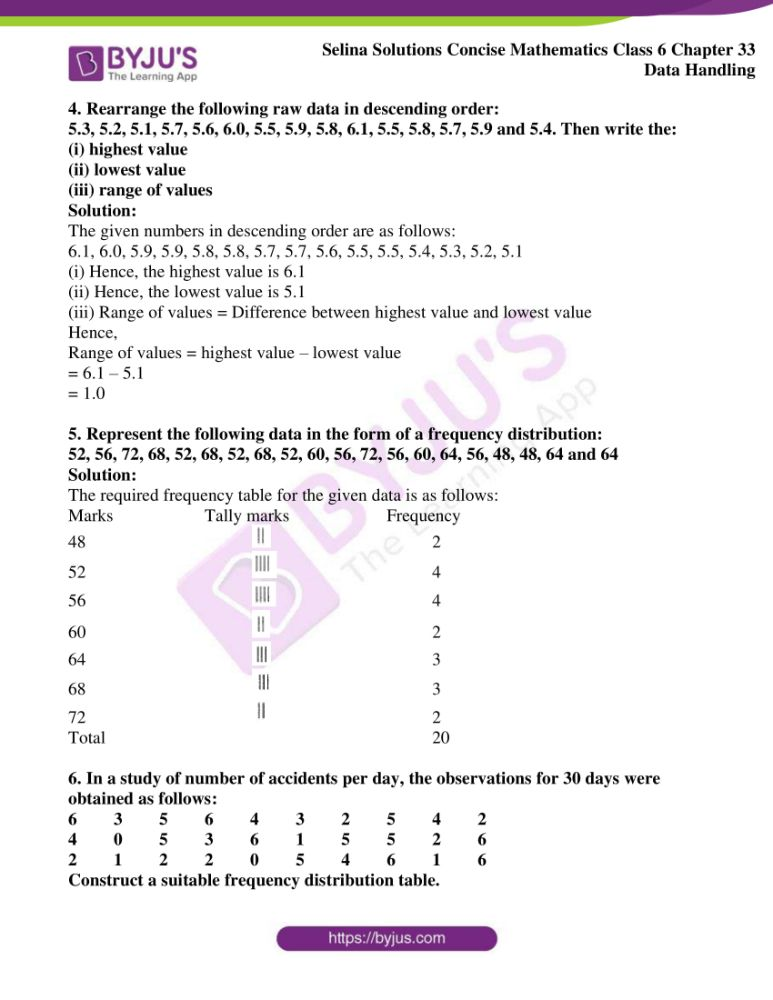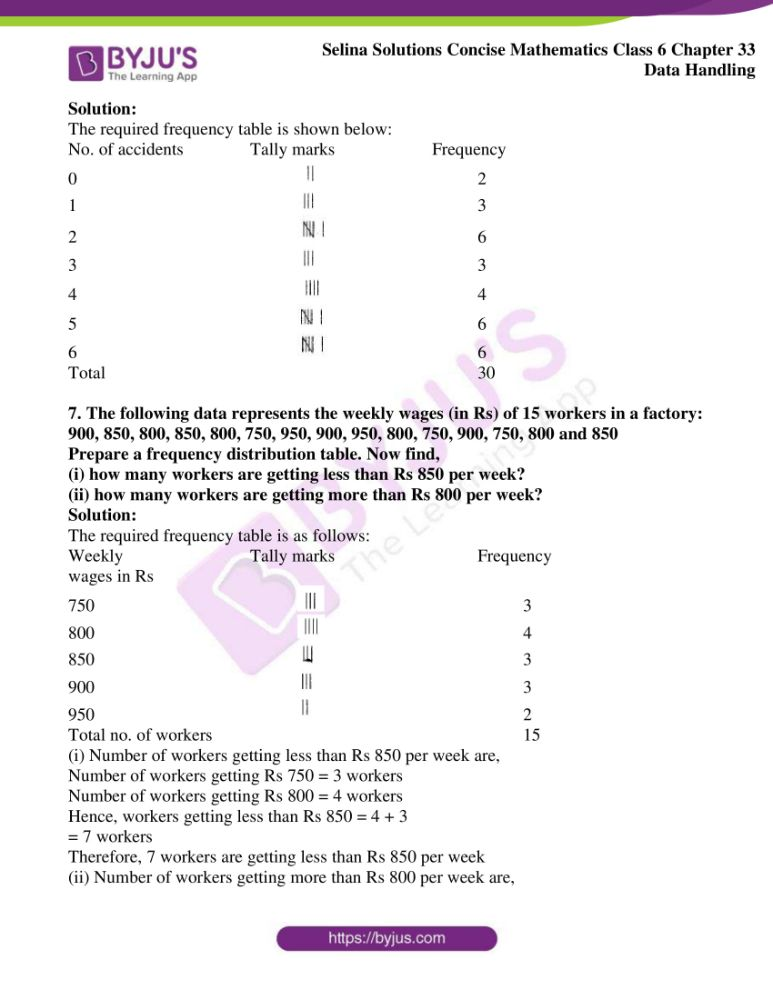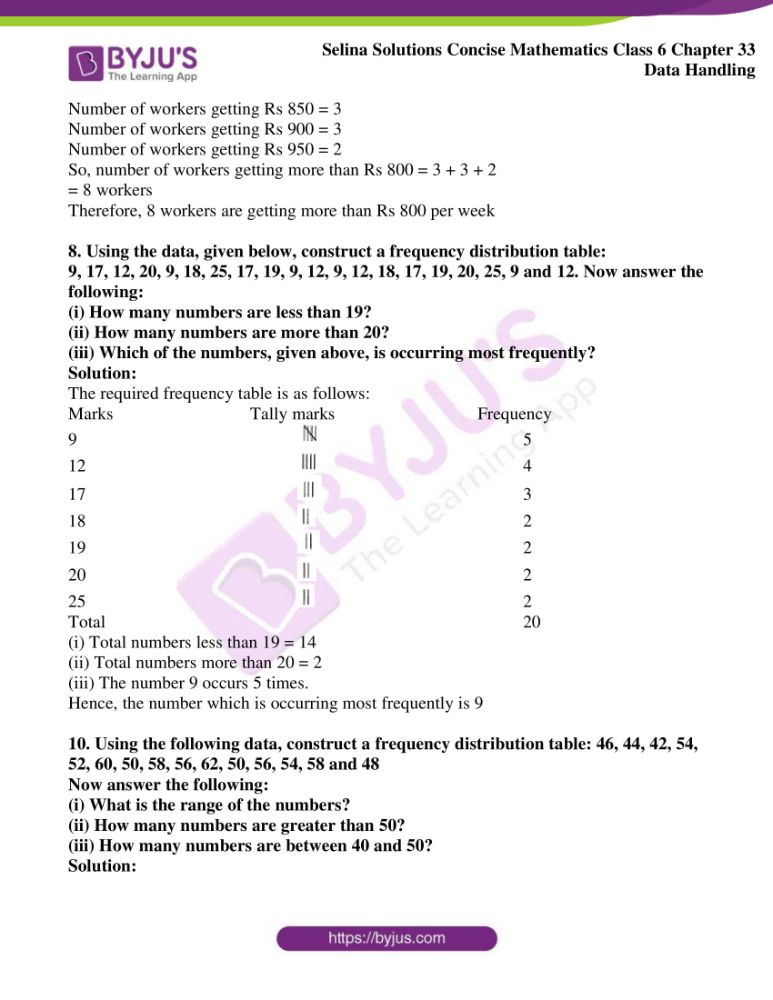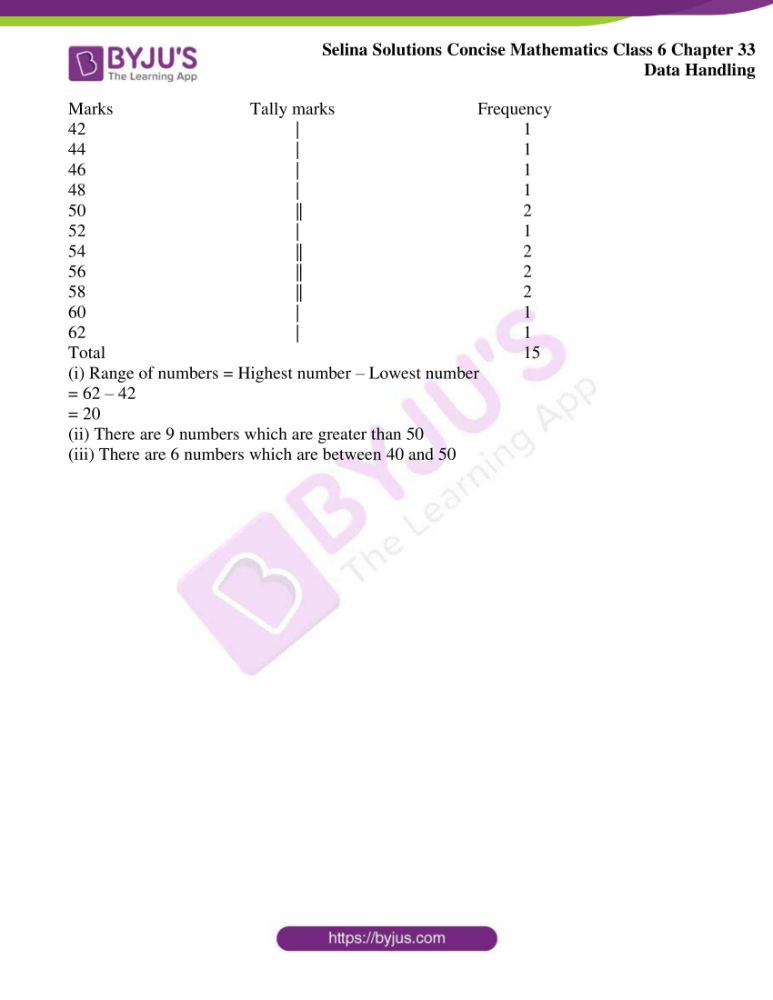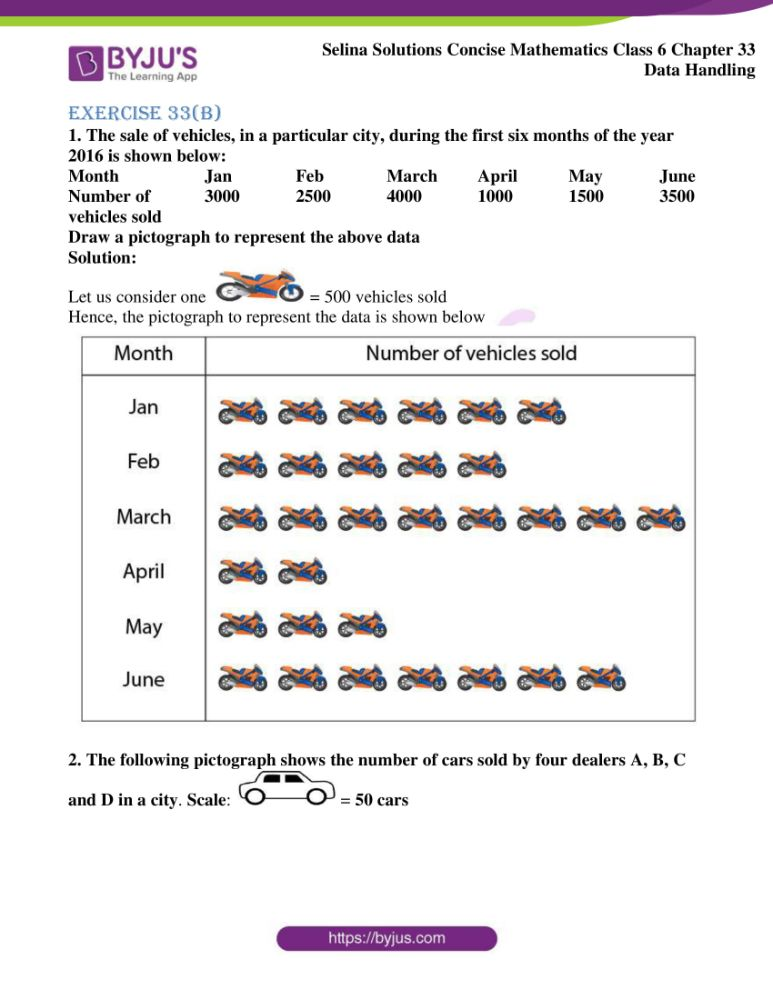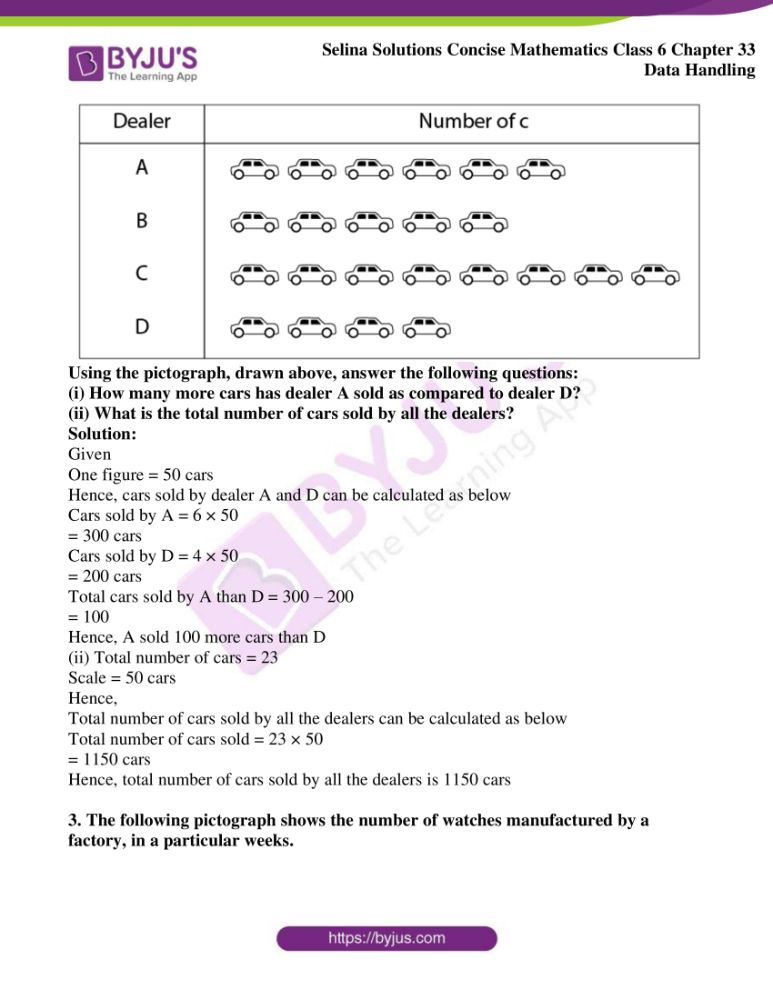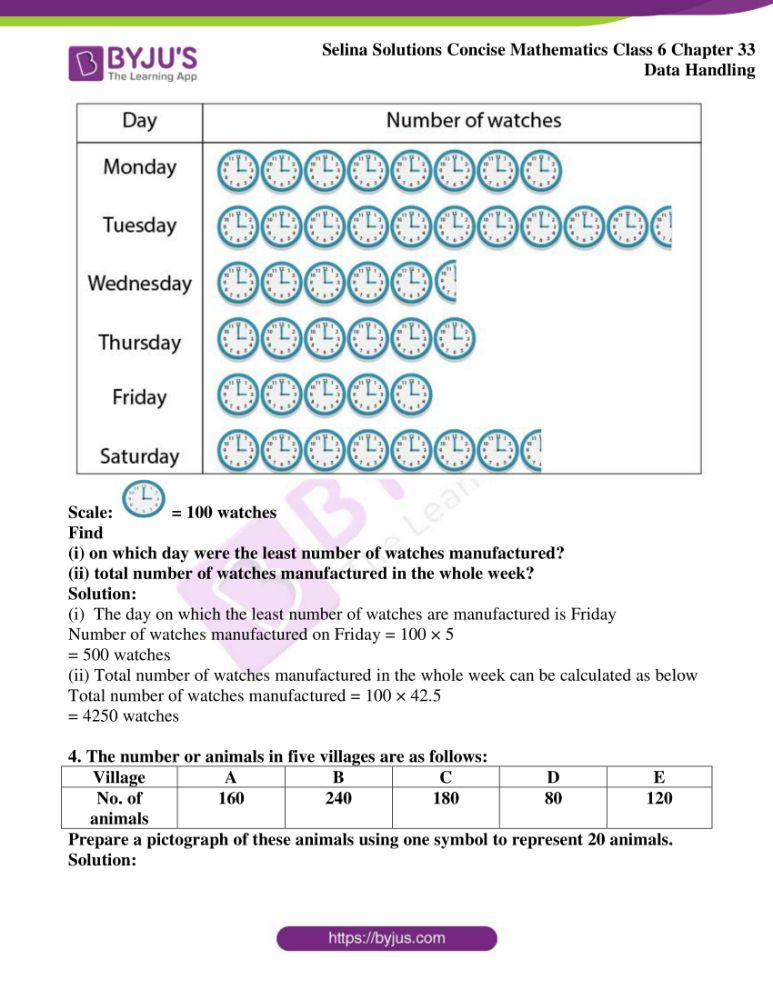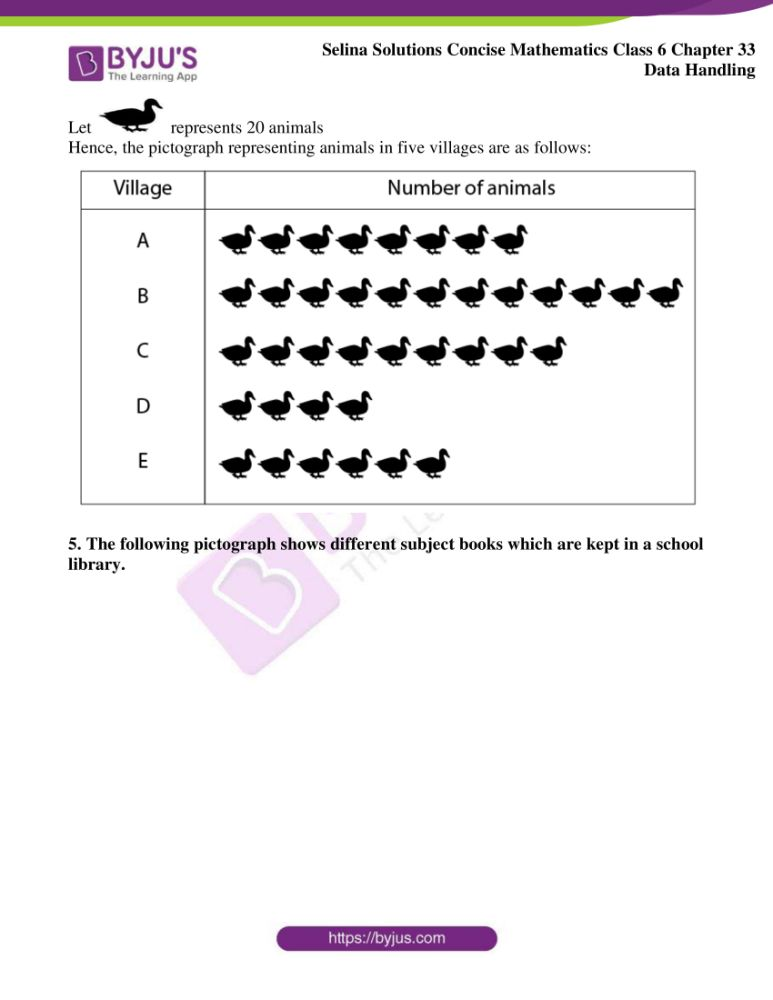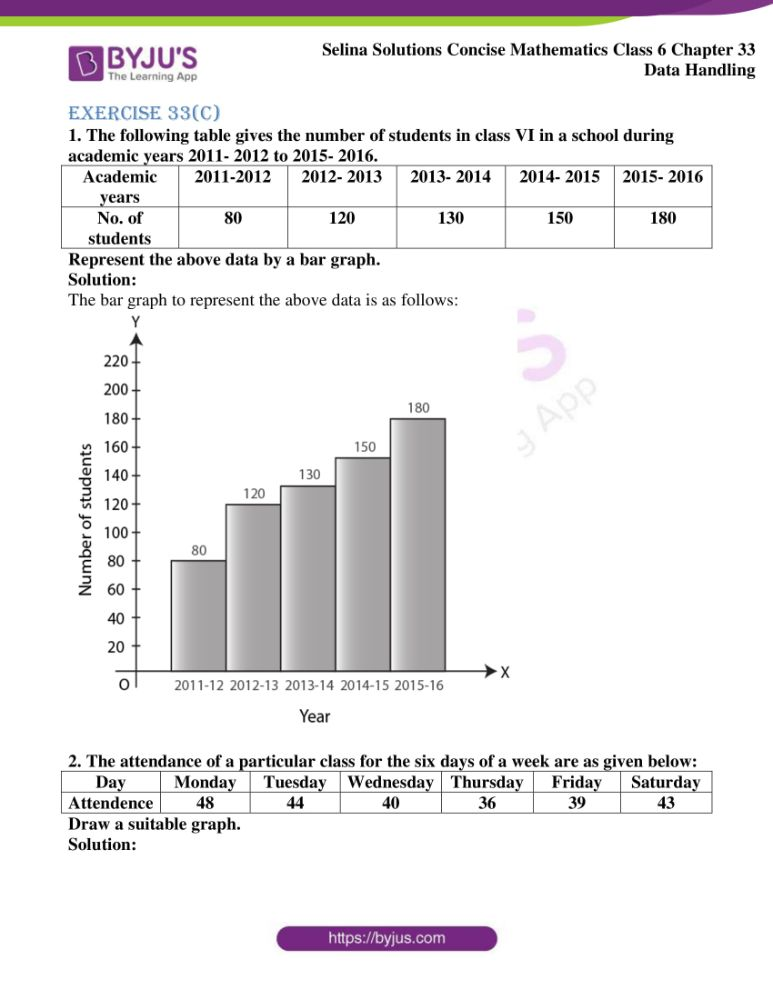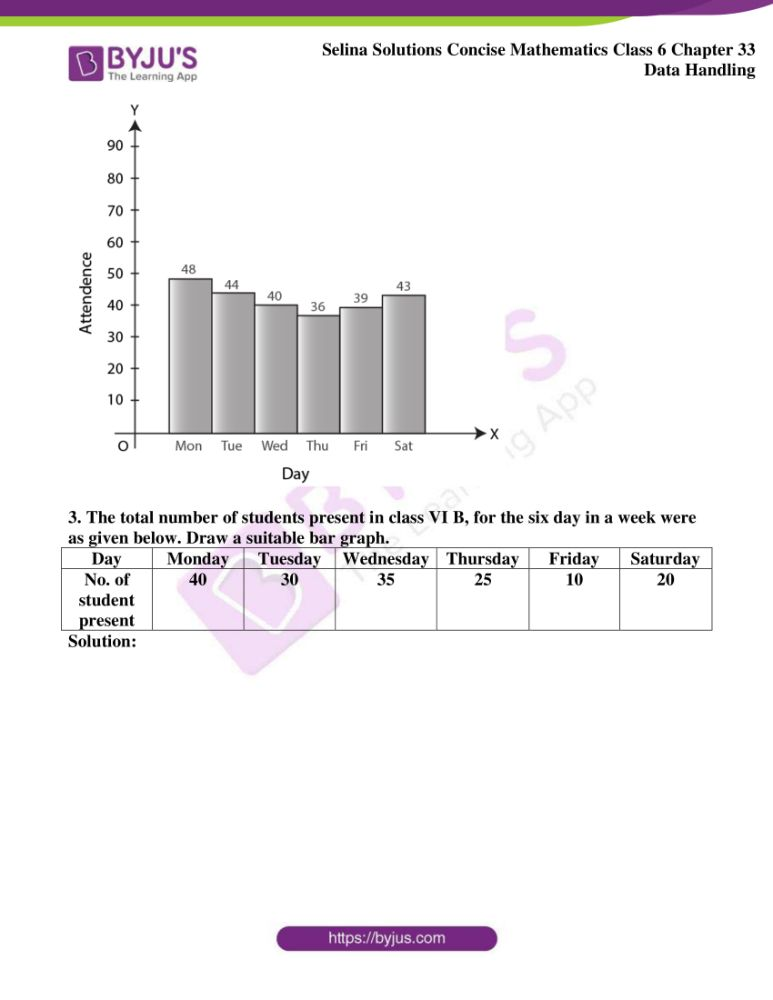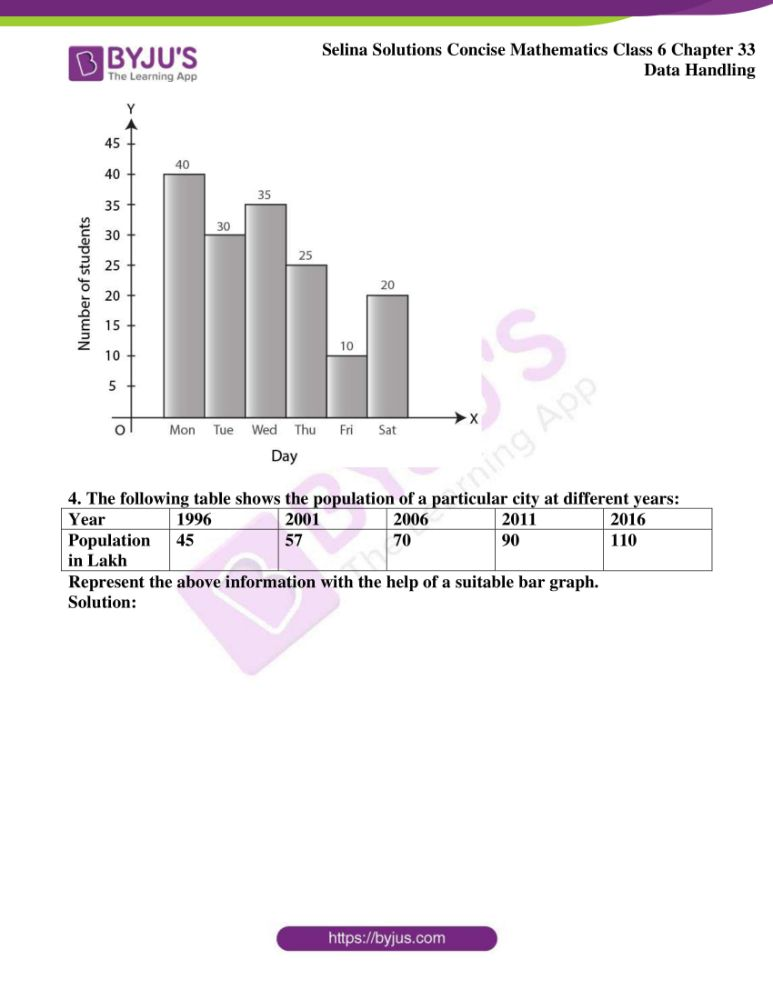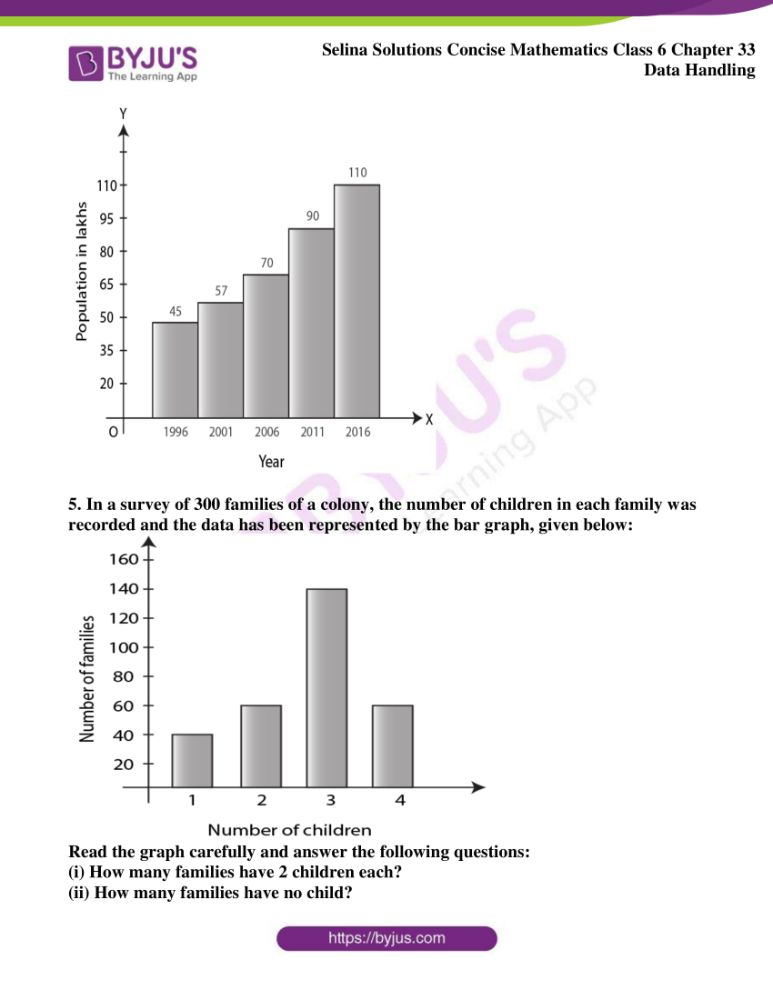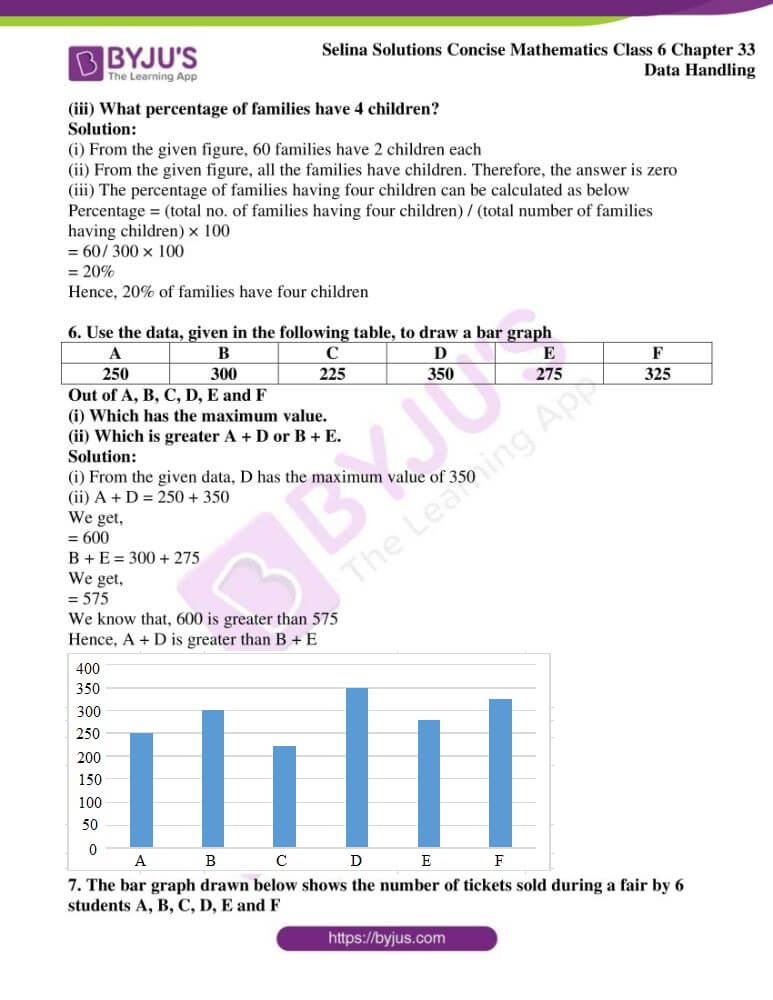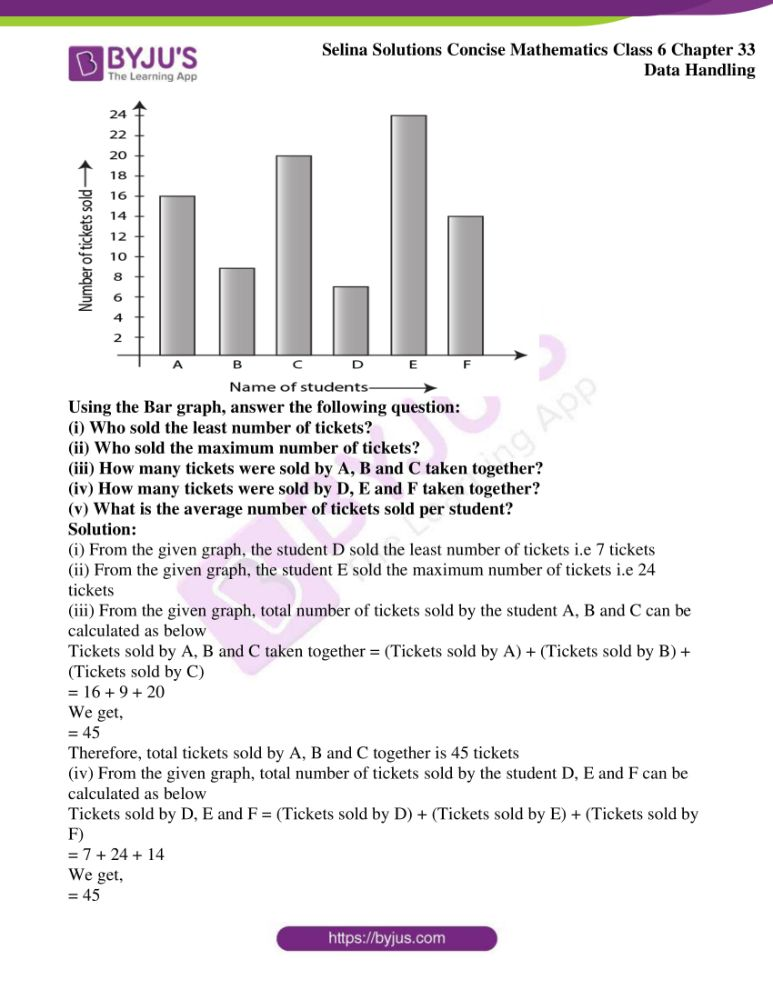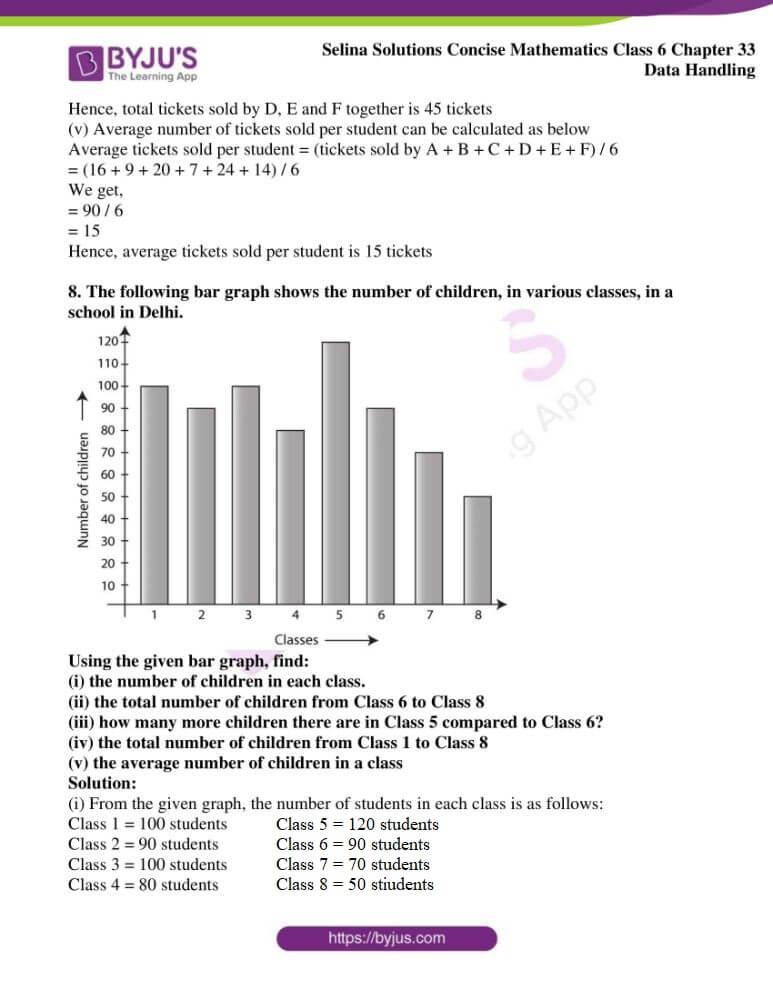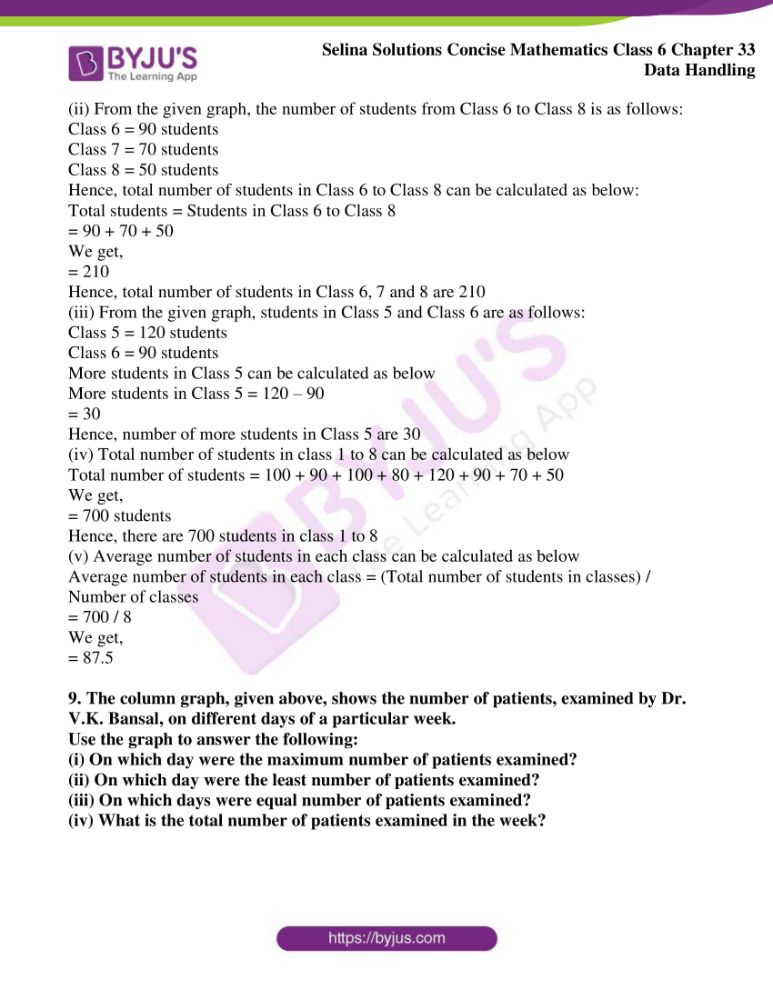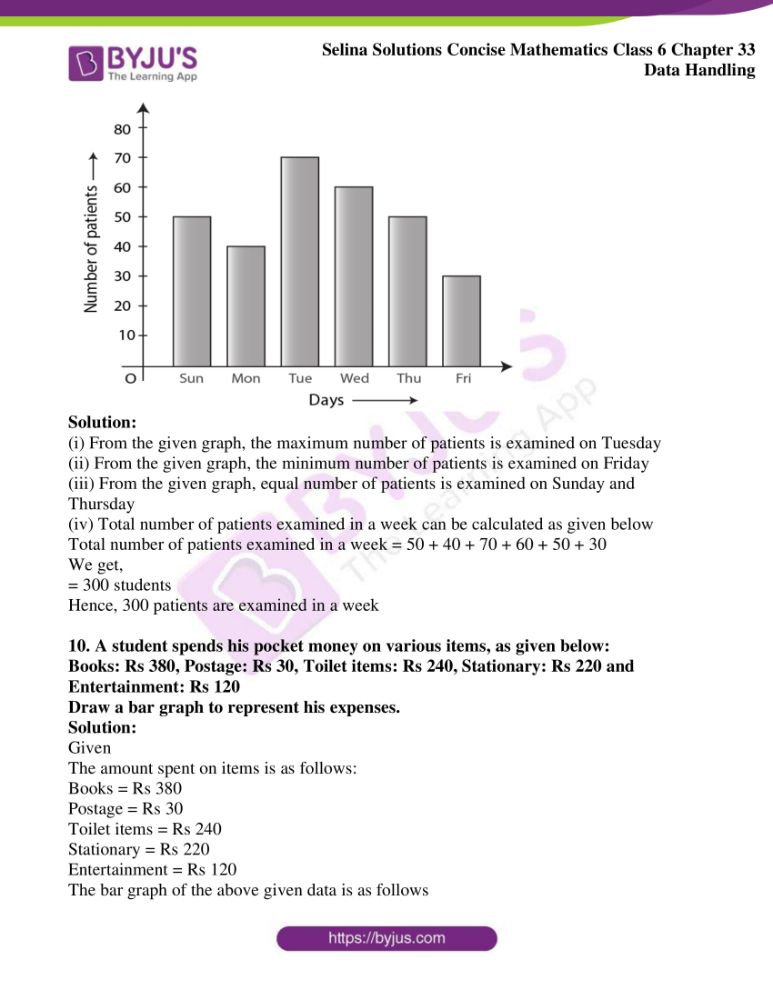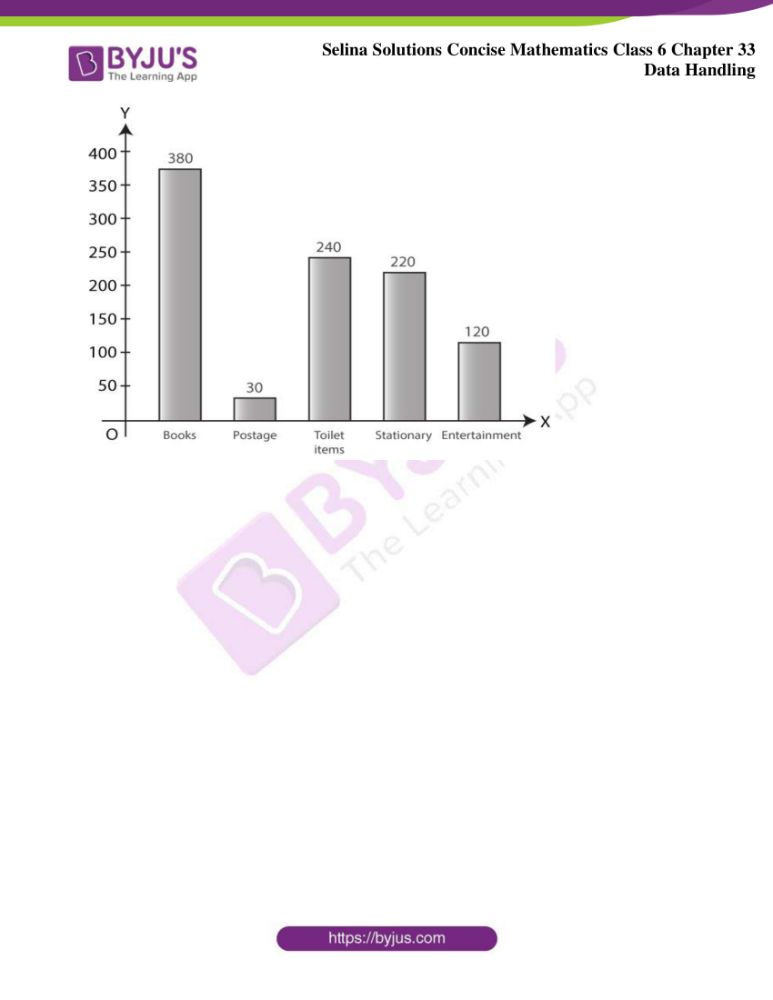### Exercises of Selina Solutions Concise Mathematics Class 6 Chapter 33 Data Handling

Exercise 33(A) Solutions

Exercise 33(B) Solutions

Exercise 33(C) Solutions

## Access Selina Solutions Concise Mathematics Class 6 Chapter 33 Data Handling

Exercise 33(A)

1.Marks scored by 30 students of class VI are as given below:

38, 46, 33, 45, 63, 53, 40, 85, 52, 75, 60, 73, 62, 22, 69, 43, 45, 33, 47, 41, 29, 43, 37, 49, 83, 44, 55, 22, 35 and 45. State:

(i) the highest marks scored

(ii) the lowest marks scored

(iii) the range of marks

Solution:

(i) The highest marks scored is 85

(ii) The lowest marks scored is 22

(iii) Range of marks = Difference between highest and lowest marks

= 85 – 22

= 63

Therefore, the range of marks is 63

2. For the following raw data, form a discrete frequency distribution:

30, 32, 32, 28, 34, 34, 32, 30, 30, 32, 32, 34, 30, 32, 32. 28, 32, 30, 28, 30, 32, 32, 30, 28 and 30

Solution:

The required frequency table is shown below

Marks    Tally marks    Frequency

28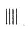4

30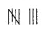8

32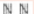10

34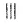3

Total                                    25

3. Define:

(i) data

(ii) frequency of an observation

Solution:

(i) Data: Information in the form of numerical figures is known as data

(ii) Frequency of an observation: The number of times a particular observation occurs is known as its frequency

4. Rearrange the following raw data in descending order:

5.3, 5.2, 5.1, 5.7, 5.6, 6.0, 5.5, 5.9, 5.8, 6.1, 5.5, 5.8, 5.7, 5.9 and 5.4. Then write the:

(i) highest value

(ii) lowest value

(iii) range of values

Solution:

The given numbers in descending order are as follows:

6.1, 6.0, 5.9, 5.9, 5.8, 5.8, 5.7, 5.7, 5.6, 5.5, 5.5, 5.4, 5.3, 5.2, 5.1

(i) Hence, the highest value is 6.1

(ii) Hence, the lowest value is 5.1

(iii) Range of values = Difference between highest value and lowest value

Hence,

Range of values = highest value – lowest value

= 6.1 – 5.1

= 1.0

5. Represent the following data in the form of a frequency distribution:

52, 56, 72, 68, 52, 68, 52, 68, 52, 60, 56, 72, 56, 60, 64, 56, 48, 48, 64 and 64

Solution:

The required frequency table for the given data is as follows:

Marks    Tally marks    Frequency

48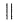2

52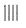4

56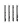4

60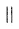2

64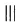3

68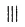3

72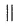2

Total                                     20

6. In a study of number of accidents per day, the observations for 30 days were obtained as follows:

6 3 5 6 4 3 2 5 4 2

4 0 5 3 6 1 5 5 2 6

2 1 2 2 0 5 4 6 1 6

Construct a suitable frequency distribution table.

Solution:

The required frequency table is shown below:

No. of accidents     Tally marks      Frequency

0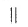2

1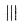3

2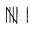6

3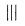3

4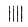4

5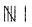6

6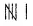6

Total                                                          30

7. The following data represents the weekly wages (in Rs) of 15 workers in a factory:

900, 850, 800, 850, 800, 750, 950, 900, 950, 800, 750, 900, 750, 800 and 850

Prepare a frequency distribution table. Now find,

(i) how many workers are getting less than Rs 850 per week?

(ii) how many workers are getting more than Rs 800 per week?

Solution:

The required frequency table is as follows:

Weekly wage (in Rs.)        Tally marks     Frequency

750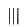3

800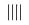4

850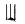3

900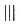3

950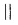2

Total no. of workers                                         15

(i) Number of workers getting less than Rs 850 per week are,

Number of workers getting Rs 750 = 3 workers

Number of workers getting Rs 800 = 4 workers

Hence, workers getting less than Rs 850 = 4 + 3

= 7 workers

Therefore, 7 workers are getting less than Rs 850 per week

(ii) Number of workers getting more than Rs 800 per week are,

Number of workers getting Rs 850 = 3

Number of workers getting Rs 900 = 3

Number of workers getting Rs 950 = 2

So, number of workers getting more than Rs 800 = 3 + 3 + 2

= 8 workers

Therefore, 8 workers are getting more than Rs 800 per week

8. Using the data, given below, construct a frequency distribution table:

9, 17, 12, 20, 9, 18, 25, 17, 19, 9, 12, 9, 12, 18, 17, 19, 20, 25, 9 and 12. Now answer the following:

(i) How many numbers are less than 19?

(ii) How many numbers are more than 20?

(iii) Which of the numbers, given above, is occurring most frequently?

Solution:

The required frequency table is as follows:

Marks        Tally marks         Frequency

9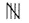5

12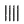4

17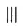3

18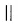2

19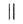2

20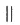2

25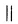2

Total                                             20

(i) Total numbers less than 19 = 14

(ii) Total numbers more than 20 = 2

(iii) The number 9 occurs 5 times.

Hence, the number which is occurring most frequently is 9

9. Using the following data, construct a frequency distribution table: 46, 44, 42, 54, 52, 60, 50, 58, 56, 62, 50, 56, 54, 58 and 48

(i) What is the range of the numbers?

(ii) How many numbers are greater than 50?

(iii) How many numbers are between 40 and 50?

Solution:

Marks          Tally marks         Frequency

42                         |                             1

44                         |                             1

46                         |                             1

48                         |                             1

50                        ||                            2

52                         |                             1

54                        ||                            2

56                        ||                            2

58                        ||                            2

60                         |                            1

62                         |                            1

Total                                                 15

(i) Range of numbers = Highest number – Lowest number

= 62 – 42

= 20

(ii) There are 9 numbers which are greater than 50

(iii) There are 6 numbers which are between 40 and 50

Exercise 33(B)

1. The sale of vehicles, in a particular city, during the first six months of the year 2016 is shown below:

Month Jan Feb March April May June

Number of 3000 2500 4000 1000 1500 3500

vehicles sold

Draw a pictograph to represent the above data

Solution:

Let us consider one= 500 vehicles sold

Hence, the pictograph to represent the data is shown below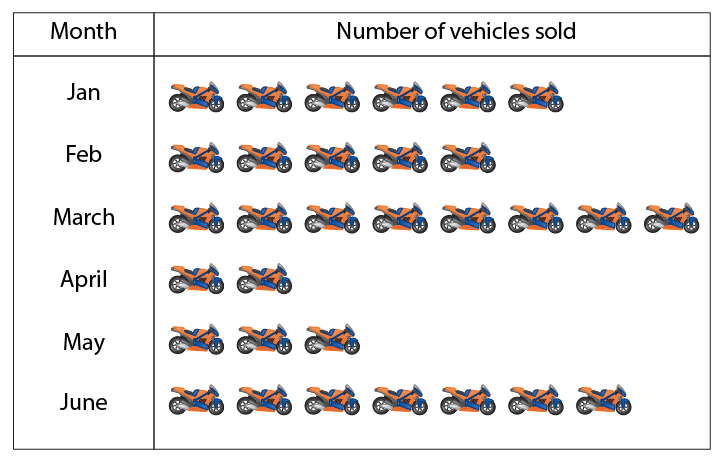2. The following pictograph shows the number of cars sold by four dealers A, B, C and D in a city. Scale:= 50 cars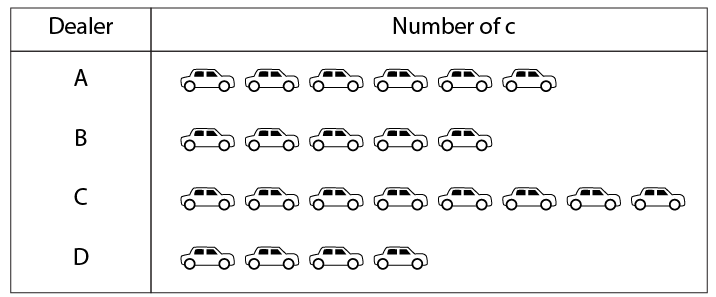Using the pictograph, drawn above, answer the following questions:

(i) How many more cars has dealer A sold as compared to dealer D?

(ii) What is the total number of cars sold by all the dealers?

Solution:

Given

One figure = 50 cars

Hence, cars sold by dealer A and D can be calculated as below

Cars sold by A = 6 × 50

= 300 cars

Cars sold by D = 4 × 50

= 200 cars

Total cars sold by A than D = 300 – 200

= 100

Hence, A sold 100 more cars than D

(ii) Total number of cars = 23

Scale = 50 cars

Hence,

Total number of cars sold by all the dealers can be calculated as below

Total number of cars sold = 23 × 50

= 1150 cars

Hence, total number of cars sold by all the dealers is 1150 cars

3. The following pictograph shows the number of watches manufactured by a factory, in a particular weeks.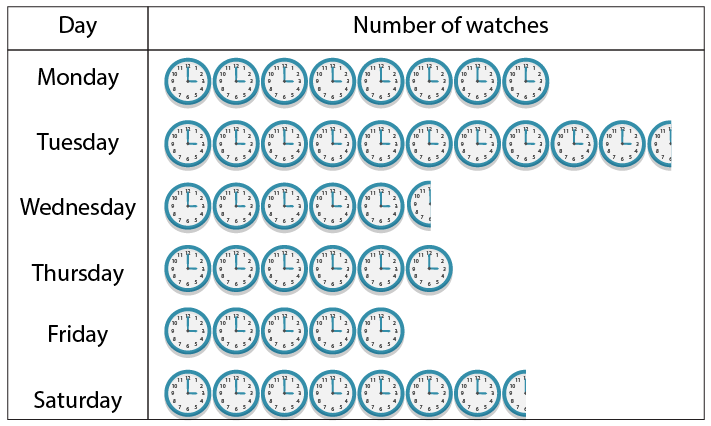Scale: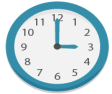= 100 watches

Find

(i) on which day were the least number of watches manufactured?

(ii) total number of watches manufactured in the whole week?

Solution:

(i) The day on which the least number of watches are manufactured is Friday

Number of watches manufactured on Friday = 100 × 5

= 500 watches

(ii) Total number of watches manufactured in the whole week can be calculated as below

Total number of watches manufactured = 100 × 42.5

= 4250 watches

4. The number or animals in five villages are as follows:

 Village A B C D E No. of animals 160 240 180 80 120

Prepare a pictograph of these animals using one symbol to represent 20 animals.

Solution:

Letrepresents 20 animals

Hence, the pictograph representing animals in five villages are as follows: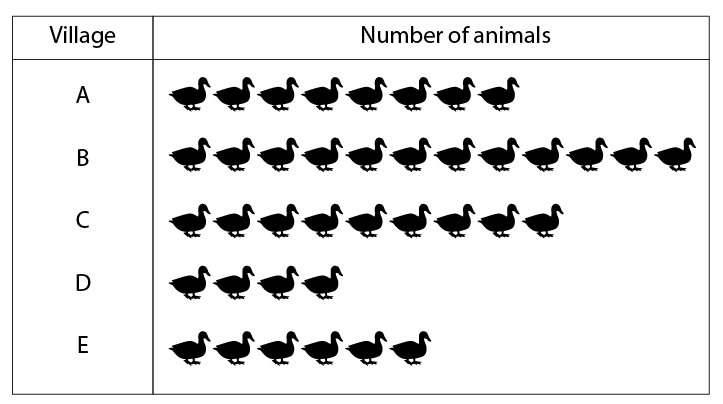5. The following pictograph shows different subject books which are kept in a school library.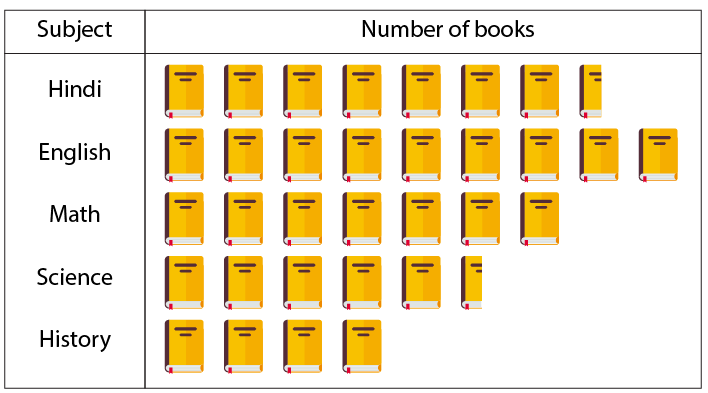Taking symbol of one book = 50 books, find:

(i) how many History books are there in the library?

(ii) how many Science books are there in the library?

(iii) Which books are maximum in number?

Solution:

(i) Given

One book = 50 books

Hence, total number of History books can be calculated as below

Total number of History books = 50 × 4

= 200 books

Therefore, there are 200 History books in the library

(ii) Given

One book = 50 books

Hence, total number of Science books can be calculated as below

Total number of Science books = 50 × 5.5

= 275 books

Therefore, there are 275 Science books in the library

(iii) From the given pictograph, English books are maximum in number in the library

Given

One book = 50 books

Hence, total number of English books can be calculated as below

Total number of English books = 50 × 9

= 450 books

Therefore, there are 450 English books in the library

Exercise 33(C)

1. The following table gives the number of students in class VI in a school during academic years 2011- 2012 to 2015- 2016.

 Academic years 2011-2012 2012- 2013 2013- 2014 2014- 2015 2015- 2016 No. of students 80 120 130 150 180

Represent the above data by a bar graph.

Solution:

The bar graph to represent the above data is as follows: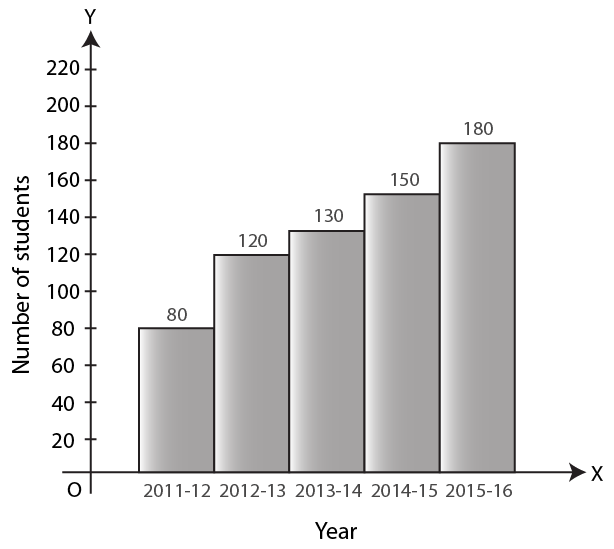2. The attendance of a particular class for the six days of a week are as given below:

 Day Monday Tuesday Wednesday Thursday Friday Saturday Attendence 48 44 40 36 39 43

Draw a suitable graph.

Solution: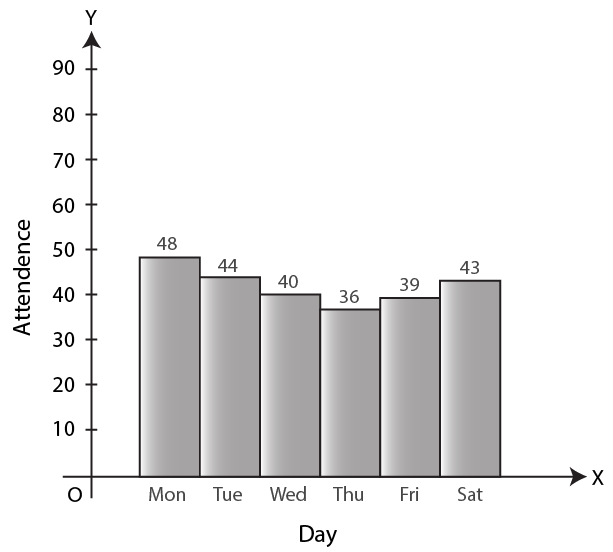3. The total number of students present in class VI B, for the six day in a week were as given below. Draw a suitable bar graph.

 Day Monday Tuesday Wednesday Thursday Friday Saturday No. of student present 40 30 35 25 10 20

Solution: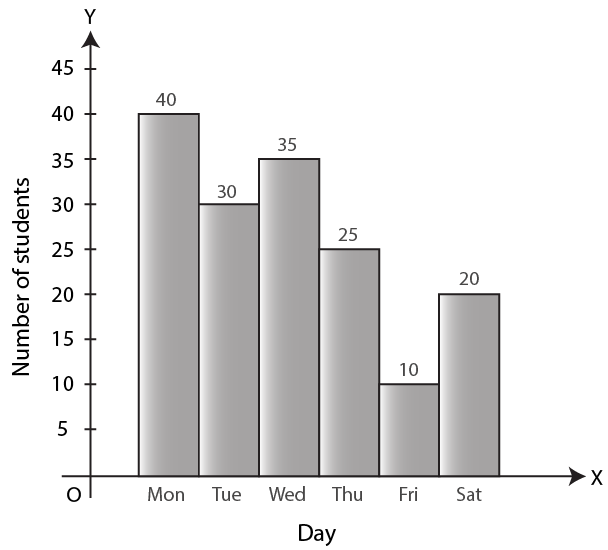4. The following table shows the population of a particular city at different years:

 Year 1996 2001 2006 2011 2016 Population in Lakh 45 57 70 90 110

Represent the above information with the help of a suitable bar graph.

Solution: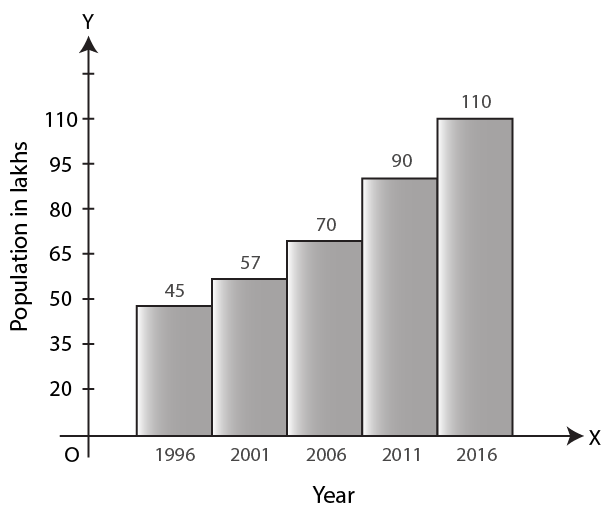5. In a survey of 300 families of a colony, the number of children in each family was recorded and the data has been represented by the bar graph, given below: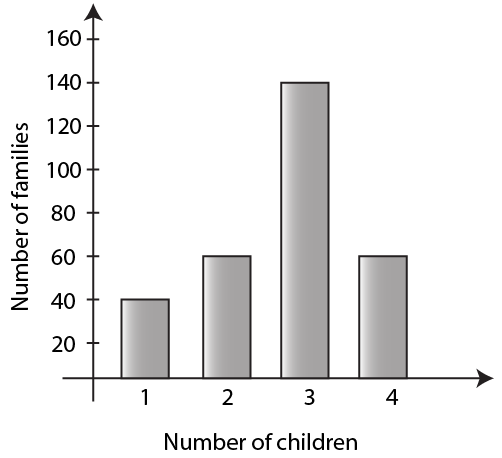(i) How many families have 2 children each?

(ii) How many families have no child?

(iii) What percentage of families have 4 children?

Solution:

(i) From the given figure, 60 families have 2 children each

(ii) From the given figure, all the families have children. Therefore, the answer is zero

(iii) The percentage of families having four children can be calculated as below

Percentage = (total no. of families having four children) / (total number of families having children) × 100

= 60 / 300 × 100

= 20%

Hence, 20% of families have four children

6. Use the data, given in the following table, to draw a bar graph

 A B C D E F 250 300 225 350 275 325

Out of A, B, C, D, E and F

(i) Which has the maximum value.

(ii) Which is greater A + D or B + E.

Solution: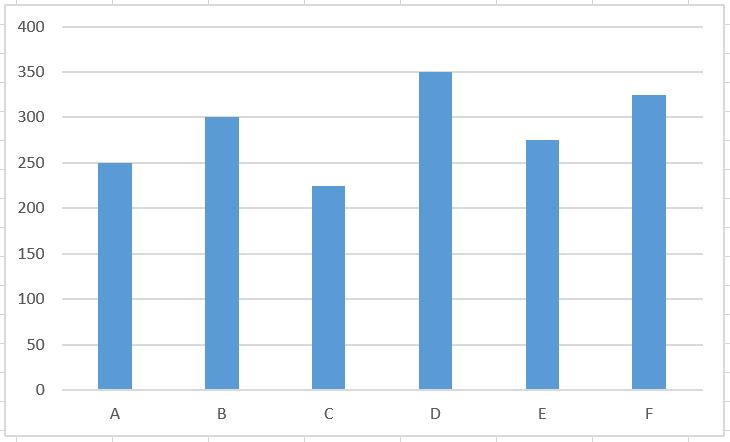(i) From the given data, D has the maximum value of 350

(ii) A + D = 250 + 350

We get,

= 600

B + E = 300 + 275

We get,

= 575

We know that, 600 is greater than 575

Hence, A + D is greater than B + E

7. The bar graph drawn below shows the number of tickets sold during a fair by 6 students A, B, C, D, E and F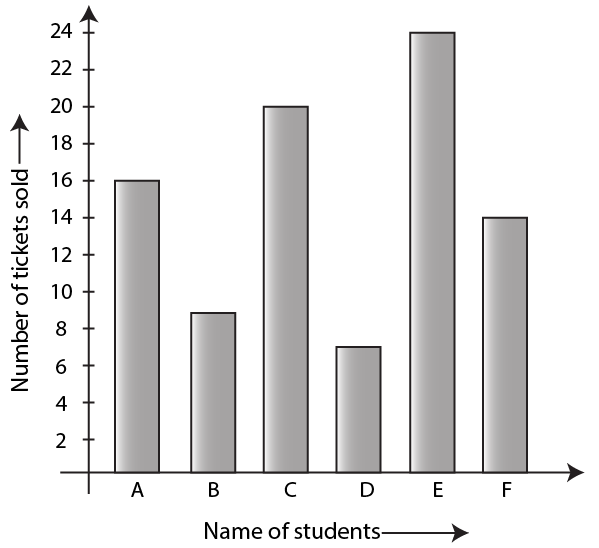Using the Bar graph, answer the following question:

(i) Who sold the least number of tickets?

(ii) Who sold the maximum number of tickets?

(iii) How many tickets were sold by A, B and C taken together?

(iv) How many tickets were sold by D, E and F taken together?

(v) What is the average number of tickets sold per student?

Solution:

(i) From the given graph, the student D sold the least number of tickets i.e 7 tickets

(ii) From the given graph, the student E sold the maximum number of tickets i.e 24 tickets

(iii) From the given graph, total number of tickets sold by the student A, B and C can be calculated as below

Tickets sold by A, B and C taken together = (Tickets sold by A) + (Tickets sold by B) + (Tickets sold by C)

= 16 + 9 + 20

We get,

= 45

Therefore, total tickets sold by A, B and C together is 45 tickets

(iv) From the given graph, total number of tickets sold by the student D, E and F can be calculated as below

Tickets sold by D, E and F = (Tickets sold by D) + (Tickets sold by E) + (Tickets sold by F)

= 7 + 24 + 14

We get,

= 45

Hence, total tickets sold by D, E and F together is 45 tickets

(v) Average number of tickets sold per student can be calculated as below

Average tickets sold per student = (tickets sold by A + B + C + D + E + F) / 6

= (16 + 9 + 20 + 7 + 24 + 14) / 6

We get,

= 90 / 6

= 15

Hence, average tickets sold per student is 15 tickets

8. The following bar graph shows the number of children, in various classes, in a school in Delhi.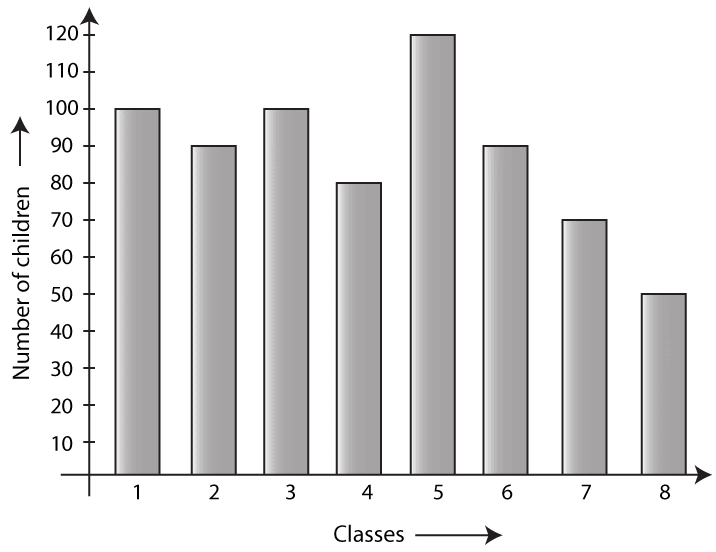Using the given bar graph, find:

(i) the number of children in each class.

(ii) the total number of children from Class 6 to Class 8

(iii) how many more children there are in Class 5 compared to Class 6?

(iv) the total number of children from Class 1 to Class 8

(v) the average number of children in a class

Solution:

(i) From the given graph, the number of students in each class is as follows:

Class 1 = 100 students

Class 2 = 90 students

Class 3 = 100 students

Class 4 = 80 students

Class 5 = 120 students

Class 6 = 90 students

Class 7 = 70 students

Class 8 = 50 students

(ii) From the given graph, the number of students from Class 6 to Class 8 is as follows:

Class 6 = 90 students

Class 7 = 70 students

Class 8 = 50 students

Hence, total number of students in Class 6 to Class 8 can be calculated as below:

Total students = Students in Class 6 to Class 8

= 90 + 70 + 50

We get,

= 210

Hence, total number of students in Class 6, 7 and 8 are 210

(iii) From the given graph, students in Class 5 and Class 6 are as follows:

Class 5 = 120 students

Class 6 = 90 students

More students in Class 5 can be calculated as below

More students in Class 5 = 120 – 90

= 30

Hence, number of more students in Class 5 are 30

(iv) Total number of students in class 1 to 8 can be calculated as below

Total number of students = 100 + 90 + 100 + 80 + 120 + 90 + 70 + 50

We get,

= 700 students

Hence, there are 700 students in class 1 to 8

(v) Average number of students in each class can be calculated as below

Average number of students in each class = (Total number of students in classes) / Number of classes

= 700 / 8

We get,

= 87.5

9. The column graph, given above, shows the number of patients, examined by Dr. V.K. Bansal, on different days of a particular week.

Use the graph to answer the following:

(i) On which day were the maximum number of patients examined?

(ii) On which day were the least number of patients examined?

(iii) On which days were equal number of patients examined?

(iv) What is the total number of patients examined in the week?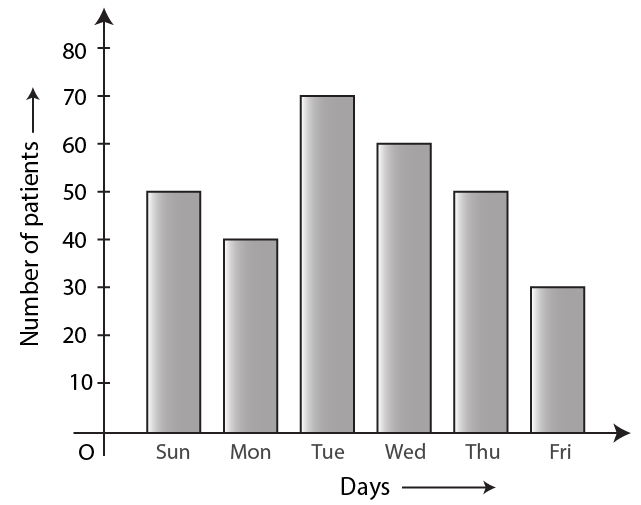Solution:

(i) From the given graph, the maximum number of patients is examined on Tuesday

(ii) From the given graph, the minimum number of patients is examined on Friday

(iii) From the given graph, equal number of patients is examined on Sunday and Thursday

(iv) Total number of patients examined in a week can be calculated as given below

Total number of patients examined in a week = 50 + 40 + 70 + 60 + 50 + 30

We get,

= 300 students

Hence, 300 patients are examined in a week

10. A student spends his pocket money on various items, as given below:

Books: Rs 380, Postage: Rs 30, Toilet items: Rs 240, Stationary: Rs 220 and Entertainment: Rs 120

Draw a bar graph to represent his expenses.

Solution:

Given

The amount spent on items is as follows:

Books = Rs 380

Postage = Rs 30

Toilet items = Rs 240

Stationary = Rs 220

Entertainment = Rs 120

The bar graph of the above given data is as follows